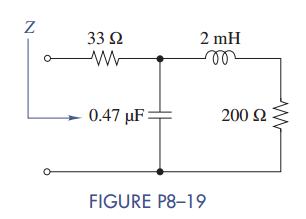### Create an Account

Home / Questions / Find the equivalent impedance Z in Figure P8–19 when ω = 50 krad/s What two elements R L ...

# Find the equivalent impedance Z in Figure P8–19 when ω = 50 krad/s What two elements R L and/or C could be used to replace the phasor circuit

Find the equivalent impedance Z in Figure P8–19 when ω = 50 krad/s. What two elements (R, L, and/or C) could be used to replace the phasor circuit?Jun 15 2020 View more View LessSubscribe To Get Solution